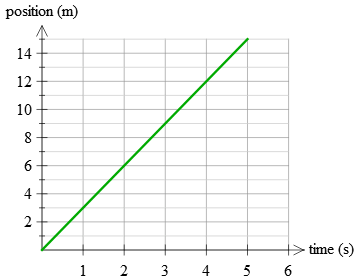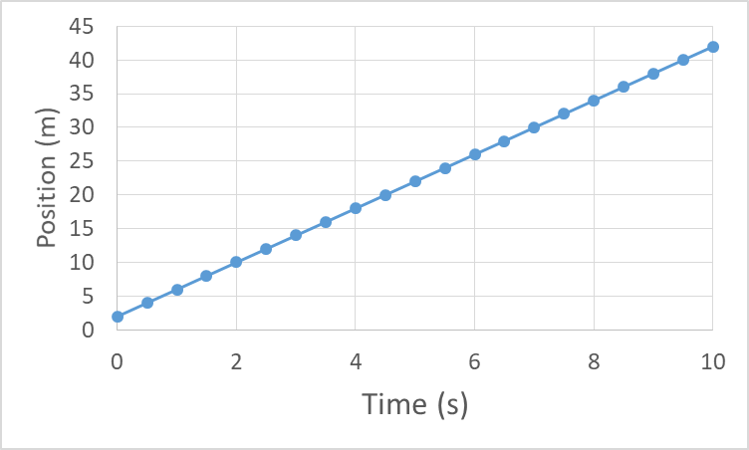# Position vs. Time Graph Study Guide

Many people are afraid of graphs and become uneasy when they have to use them. Position graphs are not terrifying creatures. They can be attractive, and they are an effective way to visually express a large quantity of information regarding an object’s motion in a little space. Let’s take a closer look at the position graphs and their characteristics.

## What is the Position Time Graph?

• Definition of position can be stated as the location of an object at any given time.
• We should be able to define the position of any item in order to explain its motion.
• The position vs. time graph, which lets us describe the motion of an object, is among the most basic types of graphs in mechanics.
• In such graphs, the vertical axis represents the object’s location, and the horizontal axis represents the time spent.
• We can examine the trajectory and position of the object exactly by interpreting a position-time graph.Position-time graph represents the position x of a particle on the y-axis and the time t on the x-axis. Graphs in the x-y plane can be drawn by keeping the following in mind.

• On the x-axis, always include an independent variable.
• On the y-axis, dependent variables must be represented.
• The dependent variable is reliant on the independent variable due to a mathematical function.

### SLOPE OF A POSITION TIME GRAPH

• The gradient of a position vs. time graph represents the velocity of the object.

• As a result, the value of the slope at a given moment shows the object’s velocity at that moment.

• The speed is determined by the numeric values of the tangent’s slope.

• Another thing to remember is that the instantaneous velocity is determined by the slope of a position vs. time graph at a given point in time.

• You may get the average velocity between two distinctive points in time by computing the mean slope between them.

• The average velocity does not have to match the instantaneous velocity.

• If, on the other hand, the slope remains constant over time, the instantaneous velocity equals the average velocity between two points.• The graph above is an example of a position vs. time graph.
• Here, the velocity of the object in motion from 0 to 5 seconds will be 5 √2 m/s.
• In between the time frame of 5 to 10 seconds, the instantaneous velocity will be equal to the average velocity as the gradient here remains constant.

###Description of Position Time Graph for Uniform Motion

The slope of a position-time graph with a time axis equals the velocity of an item moving uniformly. Therefore, the significance of the position-time graph of uniform motion is derived from the fact that its slope provides the axis’ velocity. A body moving uniformly has a slope that remains constant during its motion on the position-time graph. This suggests that the body in such a motion is moving at a constant velocity. The slope of the s-t graphs of various bodies can be used to compare the velocities of those bodies.

###Explanation of Position Time Graph for Uniformly Accelerated Motion

A body’s distance travelled in a second increases uniformly in a uniformly and positively accelerated motion. Throughout its motion, a body’s acceleration remains constant and is directed along its direction of motion. The slope of a non-straight line graph can be determined by drawing a tangent at the desired point on the graph and calculating the slope of the tangent using the standard method. When tangents are drawn at various points, the slope of the above graph increases as we move away from the origin towards the right. This indicates that the body’s velocity increases as it moves.

### Summary

• Position can be defined as the location of an object at any given time
• A position vs time graph depicts motion by plotting position relative to the starting point on the y-axis and time on the x-axis.
• The velocity is represented by the gradient of a position vs time graph. The faster the motion changes, the steeper the slope.

## FAQs

1. How do you graph a position vs. time graph?

A position vs. time graph can be plotted by determining the time of the motion on the x-axis and the position on the y-axis.

2. Why is position vs. time graphs important?

Position vs. time graphs is important as we can examine the trajectory and position of the object precisely using this.

3. What does the slope of position vs. time graph mean?

The gradient/slope of a position vs. time graph represents the velocity of the object.

We hope you enjoyed studying this lesson and learned something cool about Position vs. Time Graph! Join our Discord community to get any questions you may have answered and to engage with other students just like you! We promise, it makes studying much more fun!😎

]]>